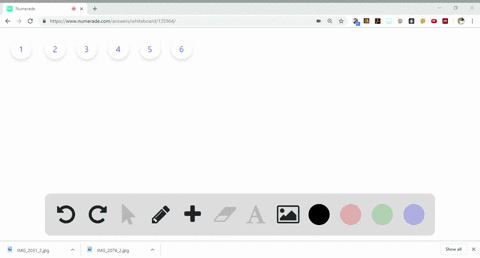Sign up for our free STEM online summer camps starting June 1st!View Summer Courses### Balance the following equations: $$\begin{arra… 18:00University of New Mexico Need more help? Fill out this quick form to get professional live tutoring. Get live tutoring Problem 13 Balance the following equations:$$
\begin{array}{l}{\text { (a) } \mathrm{Al}_{4} \mathrm{C}_{3}(s)+\mathrm{H}_{2} \mathrm{O}(l) \longrightarrow \mathrm{Al}(\mathrm{OH})_{3}(s)+\mathrm{CH}_{4}(g)} \\ {\text { (b) } \mathrm{C}_{5} \mathrm{H}_{10} \mathrm{O}_{2}(l)+\mathrm{O}_{2}(g) \longrightarrow \mathrm{CO}_{2}(g)+\mathrm{H}_{2} \mathrm{O}(g)} \\ {\text { (c) } \mathrm{Fe}(\mathrm{OH})_{3}(s)+\mathrm{H}_{2} \mathrm{SO}_{4}(a q) \longrightarrow \mathrm{Fe}_{2}\left(\mathrm{SO}_{4}\right)_{3}(a q)+\mathrm{H}_{2} \mathrm{O}(l)} \\ {\text { (d) } \mathrm{Mg}_{3} \mathrm{N}_{2}(s)+\mathrm{H}_{2} \mathrm{SO}_{4}(a q) \longrightarrow \mathrm{MgSO}_{4}(a q)+\left(\mathrm{NH}_{4}\right)_{2} \mathrm{SO}_{4}(a q)}\end{array}


a) See explanation for solution.
b) See Explanation
c) See explanation for solution.
d) See explanation for solution.

## Discussion

You must be signed in to discuss.

## Video Transcript

ah, balance chemical equation is concerned about the psychometric off reaction in a balance chemical equation. If there is a few number off moons off any element on react inside, then the same amount off moves off that Adam must be de presented on the product side for Option E. First off, all we multiply e l. Which three buy food to balance the number off aluminum atom. For the words we multiply ch four with three to Allen's big number off carbon atoms towards the board side. Off equation. Finally, we multiply water molecule by 12 to balance the hydrogen atoms in the equation for option B. Carbon, hydrogen and oxygen atoms are not balance. Let us begin with carbon atom, their fight carbon atom on the react inside, while the product sight only has one. So we multiply sti or two on the product side by five sickened. We multiply of water molecules on the broad outside by five to balance off the hydrogen atom, and lastly, we multiply oxygen or two by 13 for Option C. We know F E, which three we multiplied that by two to balance the number off Iran atoms for the words with multiply edge to s 04 by three to balance the number off cell for Adam. And finally to balance hydrogen and oxygen, we multiply that stool with six for auction d we multiply M g s 04 by three to balance the magnesia matter, Then we multiply x to s 04 by with four to balance the sulfur and oxygen atoms giving us the final equation.